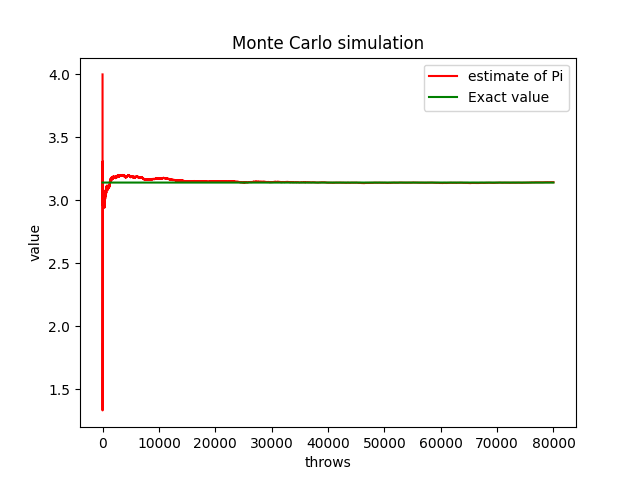# Estimating Pi…

We will be exploring a very interesting, and simple for that matter, application of statistics to help us estimate the value of Pi.

For this method, we will be imagining a simple scenario. Imagine for a moment, that we have the unit circle, inside a square.

By having the unit circle, we immediately figure out that the area of the square will be four since the radius of the circle is defined at one, which means that our square will have sides with a value of two. Now here’s where things get interesting, if we take the ratio of both of areas, we end up getting the following:

$$\frac{{{A_c}}}{{{A_s}}} = \frac{{\pi {r^2}}}{{{{\left( {2r} \right)}^2}}} = \frac{\pi }{4}$$

Both of these geometric figures end up having a ratio of pi over four between them, which is an important value for our next step in which we use a bit of imagination.

For a moment, imagine that you have a circle inside a square on the ground; suppose it starts raining. Some drops will most likely fall inside the circle and others will likely fall inside the square but outside the circle. Using this concept is how we will code our estimator pi, throwing some random numbers into the unit circle equation, as shown below:

$$x^2+y^2=1$$

Furthermore, taking a ratio of throws that landed inside our circle and the total number of throws, we can then formulate the following:

$$\frac{{N{\text{of throws inside circle}}}}{{N {\text{of total throws}}}} = \frac{{{N_C}}}{{{N_T}}}$$

And by combining our ratio between the unit circle with the square with this new equation, we can assume the next equation

$$\frac{{{N_C}}}{{{N_T}}} \simeq \frac{\pi }{4} \Leftrightarrow \frac{{4{N_C}}}{{{N_T}}} \simeq \pi$$

With this equation, we can finally start coding our estimator and see how close we can get to pi’s actual value.

The code

import matplotlib.pyplot as plt
import numpy as np
import time as t
from progress.bar import Bar

tin = t.time()

# numeri di punti della simulazione
n=80000

# Vettore coordinate  x e y dei punti casuali
x = np.random.rand(n)
y = np.random.rand(n)

Pi_Greco = np.zeros(n)
#Vettore distanza

d= (x**2 + y**2)**(1/2)

Ps = 0
Pq = 0

bar = Bar('Processing', max=n)

for i in range(n):
Pq = Pq + 1
if d[i] < 1:
Ps = Ps+1
Pi_Greco [i] = 4*(Ps/Pq)
bar.next()

bar.finish()

Pi_Greco_Reale = np.ones(n)*np.pi

tfin = t.time()

print('Valore di Pi Greco: ', 4*Ps/Pq)
print('elapsed time: ', round(tfin-tin, 3))

plt.figure(1)
plt.plot(Pi_Greco, 'red', label='estimate of Pi')
plt.plot(Pi_Greco_Reale, 'green', label='Exact value')

plt.xlabel('throws')
plt.ylabel('value')
plt.title('Monte Carlo simulation')

plt.legend()
plt.show()Results for our pi estimation. Pi is represented by the green line, while red represents our estimations.

It’s quite interesting to see our estimation start with low accuracy but as we increase our attempts, we start to get a convergence on the the value of pi, as shown by our green line. Statistical methods like this, and other more complex versions, are nice tools to understand and experiment within the world of physics. I highly recommend taking a look into some Statistical Mechanic concepts to see the beauty behind the application of statistics and probability in physics, and maybe take some time play with these concepts in Python!